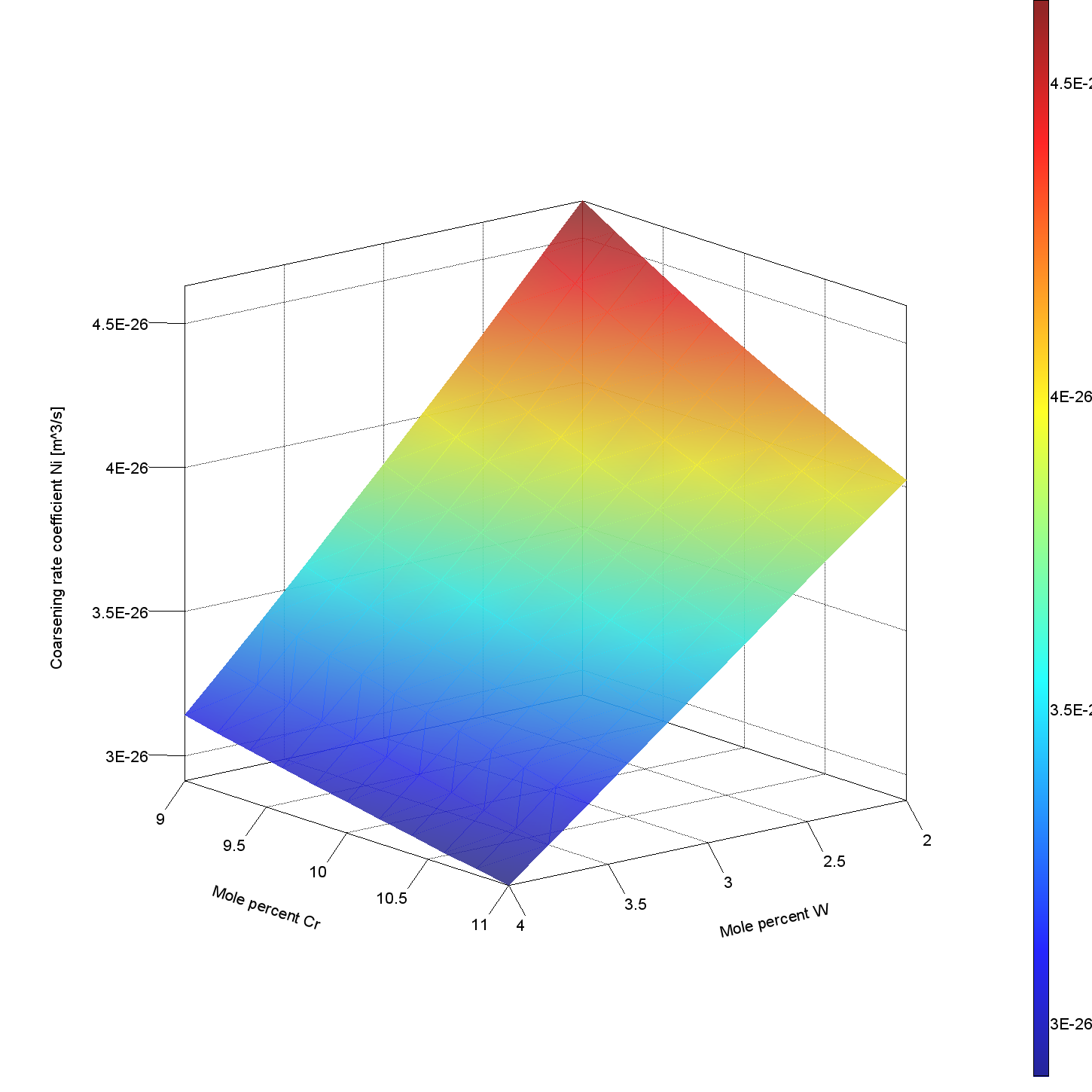# Nickel Properties

## Nickel Model Library

A set of Property Models designed to help experts working with nickel quickly and easily set up calculations using the Property Model Calculator.

## About the Nickel Model Library

The Nickel Model Library is a package of property models used to set up calculations in the Property Model Calculator. The library includes four models intended for those working in the nickel industry:  Antiphase Boundary Energy, Coarsening, Equilibrium with Freeze-in Temperature, and Solvus for Ordered Phase.

The Nickel Model Library is available for free to all users who have licenses for the nickel databases TCNI (version 11 or newer) and MOBNI (version 5 or newer) and valid Maintenance & Support Subscription (M&SS).

## Antiphase Boundary Energy Model

The Antiphase Boundary Energy Model calculates the antiphase boundary energy for Ni-base alloys with gamma prime (γ’) as the precipitate phase. The model is based on Miodownik et al. (1995), Saunders et al. (2000), Crudden et al. (2014), and Liu et al. (2018). See references

The plot shows APBE surface energy for 111 plane as a function of composition of Ti (mole %) compared to experimental data from Vamsi et al. and Chandran et al. The calculation uses an Ni3Al1-xTix alloy at room temperature using a subset of phases for a typical Ni-base superalloy.## Coarsening Model

The Coarsening Model calculates the coarsening rate coefficient of one or several precipitate phases in a matrix phase, assuming spherical geometry of the precipitating phase(s). The model is based on Anderson et al. (1992) and Morral et al. (1994). See references.

The plot shows the calculated coarsening rate of γ’at 1000 °C for an Ni0.6Mo0.92Ta12.5Al1.83Ti10.5Cr3.3W alloy when changing the Cr and W content.## Equilibrium with Freeze-in Temperature Model

The Equilibrium with Freeze-in Temperature Model calculates equilibrium at the freeze-in temperature and evaluates the properties at a different temperature. The assumption is that diffusion and phase transformations are negligible when changing from the freeze-in-temperature and, therefore, that the phase amounts and compositions of phases are kept at all other temperatures.

The electrical resistivity due to phase interface scattering is evaluated as the scattering constant times sum of the interaction between the volume fraction of all the phases. The contribution to thermal conductivity is assumed to be related to that of electrical resistivity, following the Wiedemann-Franz law. See references.

The plot shows the lattice parameters for gamma and gamma prime plotted against experimental data from Nathal et al. It is a one axis calculation of a Ni0.6Mo0.92Ta12.5Al1.83Ti10.5Cr3.3W alloy  evaluated with a range of 20 °C to 1000 °C using the Equilibrium with Freeze-in Temperature Nickel Property Model in Thermo-Calc. The freeze-in temperature is set to 1000 °C.## Solvus for Ordered Phase Model

The Solvus for Ordered Phase – Ni Property Model calculates the solvus of ordered phases such as FCC_L12, BCC_B2, NI3TA_D0A, NI3TI_D024, TCP-phases (Sigma), and so forth. The Model chooses the correct composition set by minimizing the Gibbs energy, which means that the composition set is identified automatically by the software for you.

The plot shows the solvus temperatures for NI3TA_D0A (Delta) and BCT_D022 (Gamma double-prime) compared to experimental data from Frank et al. It was calculated using the Solvus for Ordered Phase – Ni Property Model in Thermo-Calc.## Nickel Model Library Examples

The Nickel Model Library includes three examples to help users get started. The examples are available from within the software from the Help menu > Example Files > Property models > Nickel.

• PM_Ni_01_Lattice_Parameter_of_Gamma_Gamma_Prime
• PM_Ni_02_Antiphase_Boundary_Energy_of_Gamma_Prime.tcu
• PM_Ni_03_Critical_Temperatures_Alloy_718